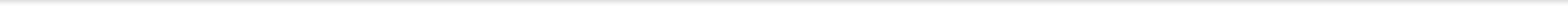Introduction

The purpose of this website to demonstrate how particle swarm optimization (PSO) techniques can be used to find an optimal design for a linear or nonlinear model. We provide MATLAB P-code for generating each of the optimal design listed on the Download page.

Quick Start

Go to the Download page to download the code into your computer, where MATLAB software is installed.

Put the p-code in a MATLAB directory, then open and activate it by typing in the command window "run" (without the double quotes) or other execution commands indicated in the Download page.

An interactive window shows up. You will see boxes for you to input the design parameters; usually these parameters are the extreme ends of the design interval for each variable, the nominal values for the model parameters and values for two PSO tuning parameters, i.e. the number of iterations and the flock or swarm size. There are recommended values already provided for these two parameters in each of the problem.

To execute the code, click “Run" on the window and the MATLAB command window shows the optimal design found iteratively at selected iteration numbers.

When the maximum number of iterations is reached, the final design is reported in the boxes in the window. Click on the “Swarm plot” to see how the swarm converges (or not) and on the “Equivalence plot” to display the directional derivative of the criterion. It this plot satisfies the equivalence theorem, the generated design is optimal among all designs on the given design interval; otherwise, it is not.

Note that variation of the interface exists but the basic idea and interpretation is the same.